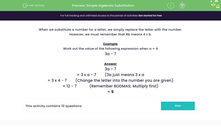# Understand Simple Algebraic Substitution

In this worksheet, students will substitute numbers for letters and work out the value of simple expressions with one variable.Key stage:  KS 3

Curriculum topic:   Algebra

Curriculum subtopic:   Substitute Numerical Values for Formulae/Expressions

Popular topics:   Algebra worksheets, Year 7 Algebra worksheets

Difficulty level:#### Worksheet Overview

When we substitute a number for a letter, we simply replace the letter with the number.

However, we must remember that 4b means 4 x b.

Example

Work out the value of the following expression when a = 4

3a - 7

3a - 7

3a just means 3 x a , so we have

3 x a - 7

Change the letter (a) into the number we've been given (4)

3 x 4 - 7

Now multiply first - remember the order of BIDMAS (or BODMAS)

12 - 7 = 5

Did that make sense?

The key to substitution in algebra is to work slowly, one step at a time.

Are you ready to have a go at some questions?### What is EdPlace?

We're your National Curriculum aligned online education content provider helping each child succeed in English, maths and science from year 1 to GCSE. With an EdPlace account you’ll be able to track and measure progress, helping each child achieve their best. We build confidence and attainment by personalising each child’s learning at a level that suits them.

Get started